Question

A piece of wire 16 m long is cut
into two pieces so that one piece
is three fifths as long as the other. Find the length of each piece.

1.The longer piece is 10 m, and the shorter piece is 6 m.
Step-by-step explanation:
16 / (5 + 3) = 2 m
2 x 5 = 10 m
2 x 3 = 6 m

2.After constructing and solving the linear equation, we obtain that the shorter piece of the wire is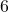m long whereas the longer piece is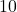m long.

### What is a linear equation?

• An equation consisting of one or more variables and constants with some mathematical operation (such as Addition, Subtraction, Multiplication, Division, etc.) between them is called a linear equation if the highest power of any variable in that equation is one.
• For example,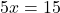is a linear equation in one variable i.e.,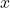whereasis a linear equation in two variablesand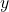.
For the given problem, we construct a linear equation and solve it to find the answer.
Let the length of the longer piece of the wire bem.
Then, by the question, the other (shorter) piece will bem long.
So, the total length will bem.
But according to the question, the total length of the wire is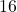m.
Thus, we must get. This is the required linear equation to be solved. By solving, we get:Also,.
Therefore, the shorter piece of the wire ism long whereas the longer piece ism long.